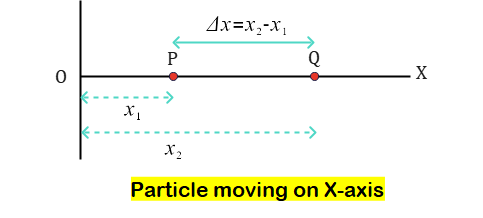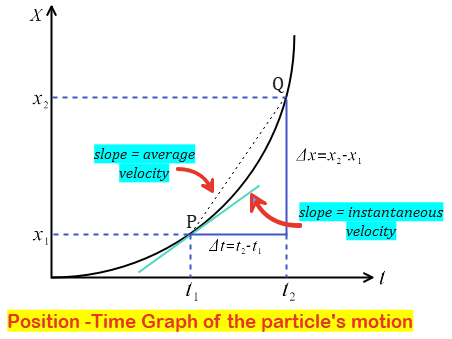# Instantaneous Velocity and Instantaneous Speed

On this page we will learn about instantaneous velocity and instantaneous speed for class 11 physics. Students preparing for competetive exams like JEE Mains, JEE Advanced and NEET can also benifit from these notes. Here we will review the concepts of instantaneous speed and instantaneous velocity as well as their formulas and units with some solved examples.

### Instantaneous Velocity and Speed in a nutshell

Instantaneous velocity is defined as the rate of change of position over a very short time interval (which almost aproaches zero). Its SI unit of measurement is m/s. The magnitude of instantaneous speed is the magnitude of instantaneous velocity. It has the same magnitude as instantaneous velocity but has no direction.

Let us now discuss what is instantaneous velocity and define it.

## Instantaneous Velocity

### Instantaneous Velocity Definition

Velocity of particle at any instant of time or at any point of its path is called instantaneous velocity.

It is important to note here that instantaneous velocity is a vector quantity having both magnitude and direction.

### Concept of Instantaneous Velocity

• To understand what is instantaneous velocity consider a particle moving along a straight line, as shown in figure 1, i.e. the particle is moving along the $X-axis$.
• In this case, the $X$ coordinate describing the motion of the particle from the origin $O$ varies with time, or we can say that the $X$ coordinate is time dependent.
•Figure 1:- Particle moving on x-axis. Image for explaining concept of instantaneous velocity.
• Again consider the figure given below and imagine second point Q being taken more and more closer to point P then calculate the average velocity over such short displacement and time interval.Figure 2:- Position time graph of the moton. Image for explaining concept of instantaneous velocity.
• Instantaneous velocity can be defined as limiting value of average velocity when second point comes closer and closer to the first point.
• Limiting value of $\frac{\Delta x}{\Delta t}$ as $\Delta t$ approaches zero is written as $dx/dt$, and is known as instantaneous velocity.Thus instantaneous velocity is $$\vec v = \lim_{\Delta t \to 0}\frac{\Delta \vec x}{\Delta t}=\frac{d\vec x}{dt}$$
• As point $Q$ approaches point $P$ in figure 2 in this limit slope of the chord $PQ$ becomes equal to the slope of tangent to the curve at point $P$.
• Thus we can say that instantaneous velocity at any point of a coordinate time graph is equal to the slope of the tangent to the graph at that point.
• From this discussion we can see that instantaneous velocity can be calculated by taking the slope of position time graph or x-t graph

### Instantaneous Velocity Formula

Instantaneous velocity formula is given by the relation

$$\vec v = \lim_{\Delta t \to 0}\frac{\Delta \vec x}{\Delta t}=\frac{d\vec x}{dt}$$
where,
• $\Delta t$ is the small time interval
• $\vec v$ is the instantaneous velocity
• $\vec x$ is the displacement of the particle in time $\Delta t$

### Unit of Instantaneous Velocity

SI unit of instantaneous velocity is $m/s$. Some other units are $ft.s^{-1}$ and $cm.s^{-1}$

## Instantaneous Speed

We know that the total distance traveled over a certain time period is equal to the total time taken divided by the total distance traveled. As this amount of time gets closer to zero, so does the distance moved.

However, the limit of the distance-time ratio is non-zero and is known as the instantaneous speed. To put it another way, instantaneous speed at any given time equals the magnitude of instantaneous velocity at that time.

### Instantaneous Speed Definition

Instantaneous speed or speed is the magnitude of the instantaneous velocity.

In contrast to average velocity and average speed, where average speed over a finite interval of time may be greater than or equal to average velocity, instantaneous speed is the magnitude of the instantaneous velocity.

### Instantaneous Speed Formula

The instantaneous speed formula is given by the relation

$$v_i=\frac{dx}{dt}$$
where,
• $dt$ is the small time interval
• $v_i$ is the instantaneous speed
• $x$ is the distance of the particle in time $dt$

### Unit of Instantaneous Speed

Unit of average velocity , average speed, instantaneous velocity and instantaneous speed is $m/s$ in SI system of units. Some other units of velocity are $ft.s^{-1}$ and $cm.s^{-1}$.

## Instantaneous Speed vs. Instantaneous Velocity

Let us now look at some of the differences between instantaneous speed and instantaneous velocity.

1. The magnitude of instantaneous velocity is given by instantaneous speed.
2. Instantaneous speed is a scalar quantity whereas instantaneous velocity is a vector quantity
3. Instantaneous velocity is defined as particle displacement divided by time at a specific instant in time. Whereas, distance times time at a particular instant of time equals instantaneous speed.

## Solved Examples

Question 1
A particle moves along x -axis and motion is given by the equation
$x= 2t + 3t^2 + 5$
where x is in meter and t in sec
Find the instantaneous velocity at t=2 sec and t=6 sec
Solution
instantaneous velocity is given by
$v= \frac {dx}{dx}$
Therefore
$v=\frac {d}{dt}(2t + 3t^2 + 5)=2+ 6t$
Now
instantaneous velocity at t=2 sec
$v_2=2+ 6(2)=14$ m/s
instantaneous velocity at t=2 sec
$v_2=2+ 6(6)=38$ m/s